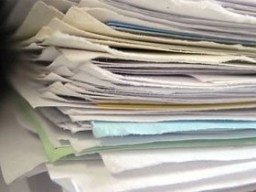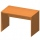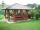# Ameeka

Ameeka is in charge of designing a school pennant for spirit week. She wants the base to be 3 1/2 feet and the height to be 6 1/2 feet. She has 20 square feet of paper available. Does she have enough paper?

Result

x=

### Step-by-step explanation:Did you find an error or inaccuracy? Feel free to write us. Thank you!#### You need to know the following knowledge to solve this word math problem:

We encourage you to watch this tutorial video on this math problem:

## Related math problems and questions:

• AlexandraAlexandra made a rectangular quilt the measured 3 1/4. 2 3/4 feet in width. What is the area of the quilt in square feet? Write an equation to solve.
• Father's gardenJohn's father has a garden in the back yard. The dimensions of the garden are shown here. A green rectangle is shown. The width is labeled as 2 and two-thirds feet. The length is labeled as 7 and three-fourths feet. Calculate the area of the garden. You f
• Rectangle areaWhat is the area of a rectangle that has a length of 4 feet and a width of 4/10 feet?
• JuilaJuila has a rectangular table that is 3 1/2 feet long and 1 3/4 feet wide. What is the area of Juila's table?
• PavilionThe rectangular pavilion with dimensions 3.5 m and 2.75 m to be paved with square tiles of side 25 cm price of CZK 22 per 1 piece or rectangular tiles with sides of 20 cm and 15 cm in the price of CZK 11 per 1 pc. Which solution is cheaper (write its pric
• Paper squaresThe paper rectangle measuring 69 cm and 46 cm should be cut into as many squares as possible. Calculate the lengths of squares and their number.
• A rectangle 6A rectangle measures 2 4/5 inches by 2 1/5 inches. What is its area?
• PresentGift box has a rectangular shape with dimensions of 8×8×3 cm. Miloslav wants to cover with square paper with sides of 18 cm. How much paper left him?
• The bookThe book has 280 pages. Each side is a rectangle with sides 15 cm and 22 cm. a) how many leaves have the book? b) at least how many square meters of paper should be used to produce this book?
• Octagon from rectangleFrom tablecloth rectangular shape with dimensions of 4 dm and 8 dm we cuts down the corners in the shape of isosceles triangles. It thus formed an octagon with area 26 dm2. How many dm2 we cuts down?
• Jared's room paintingJared wants to paint his room. The room's dimensions are 12 feet by 15 feet, and the walls are 9 feet high. Two windows measure 6 feet by 5 feet each. There are two doors, whose dimensions are 30 inches by 6 feet each. If a gallon of paint covers approxim
• Paper cuttingSusanna is cutting construction paper into rectangles for a project. She needs to cut one rectangle that is 10 inches × 14 1/2 inches. She needs to cut another rectangle that is 10 1/3 inches by 10 1/2 inches. How many total square inches of construction
• Colored paperCan a 4 cm, 3 cm and 2 cm block be glued with a colored quarter of paper measuring 7 cm and 8 cm?
• Paper boxCalculate whether 11 dm² of paper is sufficient for gluing a box without a lid with bottom dimensions of 2 dm and 15 cm and 12 cm high. Write result as: 0 = No, 1 = Yes
• MohammedMohammed grew a garden last year. His plot of land was 6 3/4 feet by 10 feet. He wants to have twice as much area and his garden this year. How much area will the new garden take up?
• The rugJosie has a rug with the area of 18 square feet. She will put the rug on the floor that is covered in 1/3 square foot tiles. How many tiles will the rug cover?
• Find the 28Find the area of a square with a side length of 4/5 yards.